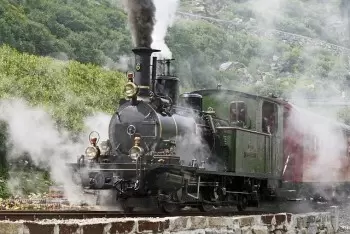# Examples of the First Law of Thermodynamics and the Conservation of EnergyThe first law of thermodynamics states: "The total energy of an isolated system is neither created nor destroyed, the amount of energy remains constant.” Energy is transformed from one form to another.

Although the definition seems very technical and challenging to understand, numerous everyday examples apply this thermodynamic principle.

We will use three examples:

• A boy who throws up a ball in the air.

• Steam machines are thermodynamic machines transferring heat frequently.

• Solar energy, especially solar thermal, experiences the conservation of energy's law.

According to the international system of units, energy, heat, work, and all forms of energy are measured in Joules.

All examples in real life are open systems. Closed systems only exist on the paper to simplify the calculations.

## Law of Conservation of Energy in a Balloon Thrown into the Air

Two types of energy are involved in this example: kinetic and potential.

1. When a boy throws a ball into the air, the ball experiences several energies transformations.

2. When the ball leaves the boy's hands, it has speed (kinetic energy). It has not yet gained height; therefore, it has no potential energy.

3. As the ball gains height, it loses kinetic energy and gains potential energy.

4. When it reaches the highest point, it only has potential energy.

5. Finally, it goes down again, and the energies are also reversed.

## Steam Machines

The development of the steam engine involved the start of the development of the first of the laws of thermodynamics. It is a thermodynamic process where heat transfer has enormous importance.

It is the first time that a thermodynamic transformation has occurred to convert thermal energy into mechanical energy. The work done by the system is based on the variation of the pressure-volume ratio.Let's analyze how energy is transformed into a steam locomotive. We consider the locomotive as a thermodynamic system.

1. Initially, all the internal energy of the system is the internal energy of the fuel.

2. When combustion, there is a change in energy; it is transformed into thermal energy.

3. All of this amount of heat is used to generate steam and power the engine's pistons. At this time, it is converted into mechanical energy.

4. The movement of the engine makes the locomotive to move. Having speed implies having kinetic energy.

In our example, the locomotive is not an isolated system. Therefore there is heat exchange with the outside. In a steam locomotive there are many losses, for instance:

• The smoke from combustion and the hot steam that escapes.

• The friction between the different mechanisms generates negative work.

• Friction with the tracks makes loose heat and work.

• Friction with air is a way through the energy escape from the system.

• Part of the heat generated in the boiler is transmitted to the air.

## Conservation of Energy in Solar Energy

An example of this principle is solar energy. It is applied both in photovoltaic and in solar thermal.

The atoms of the particles that make up the Sun contain energy (internal energy). These atoms continuously undergo a nuclear reaction. Nuclear fusion converts this chemical energy into radiation.

Solar panels capture the solar radiation that reaches Earth.

Solar panels transform this energy into electrical energy (photovoltaic energy) or heat energy (thermal energy).

### So Why Is the Performance of a Solar Panel, Not 100%?

All the solar energy that reaches the solar panel is transformed. But it is not all transformed into the same type of energy. Part of the radiation received by a photovoltaic module is converted into electricity.

However, another part is converted to heat, heating the panel; or bounces back into the atmosphere.

Author:

Published: June 4, 2020
Last review: June 4, 2020